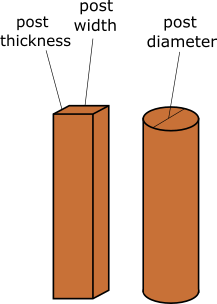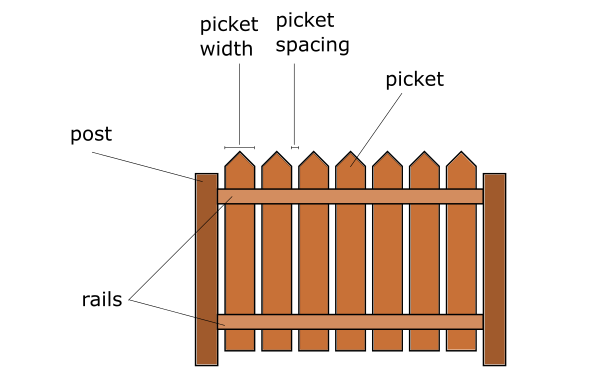# Fence Material Calculator

Created by Luis Hoyos
Reviewed by Luciano Miño
Last updated: Jun 05, 2023

Welcome to the fence material calculator, a tool created to obtain the amount of material to build the fence of your dreams.

• No matter if you selected wood fencing materials or vinyl fence materials. Calculating how much material you'll need is crucial.
• Once you've made the estimations, we recommend buying about 10% more fencing materials than calculated to correct mistakes or for future maintenance.

## How to use this fence material calculator (and the equations behind it)

### Number of posts and sections

To obtain these quantities, input the length your fence will have and the space between the posts (typically 6-8 feet or 2-3 meters).

The tool will use the following formulas:

$\scriptsize \mathrm{\#\ of\ posts = \frac{Fence\ length}{Post\ spacing} + 1} \\ \mathrm{\#\ of\ sections = \#\ of\ posts - 1}$
• If you obtain a decimal in the $\mathrm{\#\ of\ posts}$ formula (as usual), round it up to the nearest integer.
• $\text{Fence length}$ and $\mathrm{\#\ of\ posts}$ must use the same units. Our length converter can help.

### Height of the posts

To obtain this quantity, you only need to input the height of the fences. As at least 1/3 of the post must be buried in the ground, the formula is as follows:

$\scriptsize \mathrm{Post\ length = 1.5 \times Fence\ height}$

### Number of rails needed

To know the number of rails, input the rails per section. Additionally, the number of sections must also be calculated.

$\scriptsize \mathrm{\#of\ rails = Rails\ per\ section \times \#of\ sections}$

### Number of pickets needed

For the number of pickets, input the fence length, the picket width, and the picket spacing.

This is the equation behind:

$\scriptsize \mathrm{\#of\ pickets \!=\! \frac{Fence\ length}{Picket\ width \!+\! Picket\ spacing}}$
• Fence length, picket width, and spacing must have the same units. Our distance converter calculator can help.
• Like with the $\mathrm{\#\ of\ posts}$ formula, round up the $\mathrm{\#\ of\ pickets}$ to the nearest integer.

### Concrete for post footing

The geometry determines how much concrete we'll need to secure the posts. Therefore, you'll need to input the shape and dimensions of the post to obtain the concrete volume. Look at the formulas in the post hole concrete calculator.## FAQ

### How many pickets do I need for a 100 feet fence?

To know how many pickets you need for a 100 feet fence, consider the picket width and spacing:

• If you use pickets of 5½" width with a 2½" spacing, you need 150 pickets; or
• If you use pickets of 3½” width with a 2½” spacing, you need 200 pickets.
• For other widths or spacings, use our fence material calculator.

### What's the difference between a fence and a gate?

The difference between a fence and a gate is simple:

• A fence is a structure enclosing an area. Its purpose can be privacy, protection, decoration, or noise isolation.
• A door is used to access or exit an enclosed area, such as one enclosed by a fence.
Luis HoyosNumber and length of posts needed
Fence length
ft
Post space
ft
Number of posts
Number of sections
Fence height
ft
Post length
ft
Number of rails needed
Rails per section
Number of rails
Number of pickets needed
Picket width
in
Picket spacing
in
Number of pickets
Concrete for post footing
Post shape
cuboid
Post width
in
Post thickness
in
Concrete volume
cu yd
Visit the cement calculator to determine how much cement, sand, gravel, water, or money you'll need for this concrete volume.
People also viewed…

### Concrete block

Use this concrete block calculator to estimate the number of blocks needed to build a wall with specific dimensions, as well as to calculate the costs.

### River rock

Use our river rock calculator to estimate how much river rock you need for your project. You can also find out its total cost.

### Sunbathing

Do you always remember to put on sunscreen before going outside? Are you sure that you use enough? The Sunbathing Calculator ☀ will tell you when's the time to go back under an umbrella not to suffer from a sunburn!